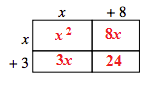Home > CC4 > Chapter 3 > Lesson 3.2.2 > Problem3-90

3-90.

What is the area of each part of the entire rectangle at right? What is the area of the whole figure? Write the area of the rectangle as a product and as a sum. Homework Help ✎

 $x$ $+8$ $x$ $+\ 3$

Copy the generic rectangle.

Fill in the table by multiplying the column and row terms.

The area could be written as a product. The RED terms are the factors for the product.

The area could be written as a sum. Add the RED terms within the rectangle.$\text{Area }=(x+8)(x+3)$

$\text{Area }=x^2+8x+3x+24=x^2+11x+24$